The Wave Equation

The wave equation for a plane wave traveling in the x direction is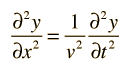where v is the phase velocity of the wave and y represents the variable which is changing as the wave passes. This is the form of the wave equation which applies to a stretched string or a plane electromagnetic wave. The mathematical description of a wave makes use of partial derivatives.

In two dimensions, the wave equation takes the formwhich could describe a wave on a stretched membrane.

 Varieties of wave equations
Index

Wave concepts

 HyperPhysics***** Sound R Nave
Go Back

Waves in Ideal String

The wave equation for a wave in an ideal string can be obtained by applying Newton's 2nd Law to an infinitesmal segment of a string.If a constant horizontal tension T is maintained in the string, then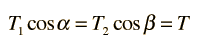Newton's 2nd law becomes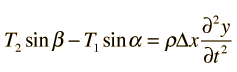Combination of these two expressions for small angles gives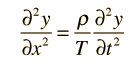Show details Solutions to wave equation
Index

Wave concepts

Reference:
Kreyzig
Ch. 9

 HyperPhysics***** Sound R Nave
Go Back

String Wave Equation Development

 Analysis of the forces on a segment of stretched string gives two relationships: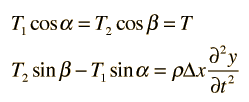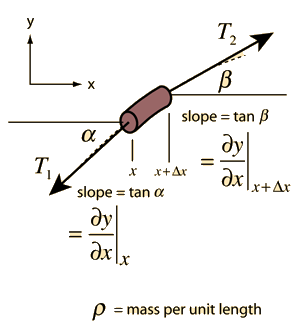Combining gives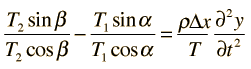and relating this to the slopes at the segment ends gives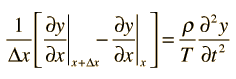In the limit Δx→ 0 this becomesWave equation
Index

Wave concepts

Reference:
Kreyzig
Ch. 9

 HyperPhysics***** Sound R Nave
Go Back

Ideal String Constraints

In order for the wave equation to apply to the waves in a string, it must meet certain constraints. For an ideal string, it is assumed that

1. The string is perfectly uniform with a constant mass per unit length, and is perfectly elastic with no resistance to bending.

2. The string tension is presumed to be large enough so that gravity can be neglected.

3. Small segments of the string are presumed to move transversely in a plane perpendicular to the string, and that the displacements and slopes of segments of the string are small.

Though stringent, these idealizations permit the development of a wave equation which describes well the vibrations of thin real strings.

Index

Wave concepts

 HyperPhysics***** Sound R Nave
Go Back